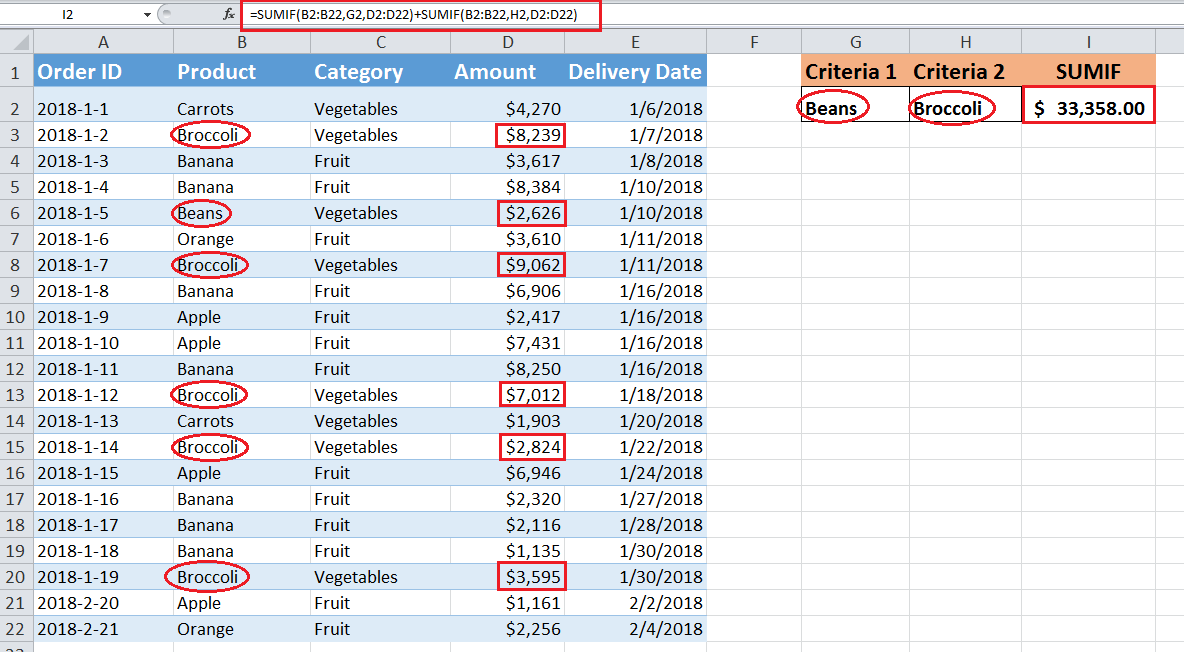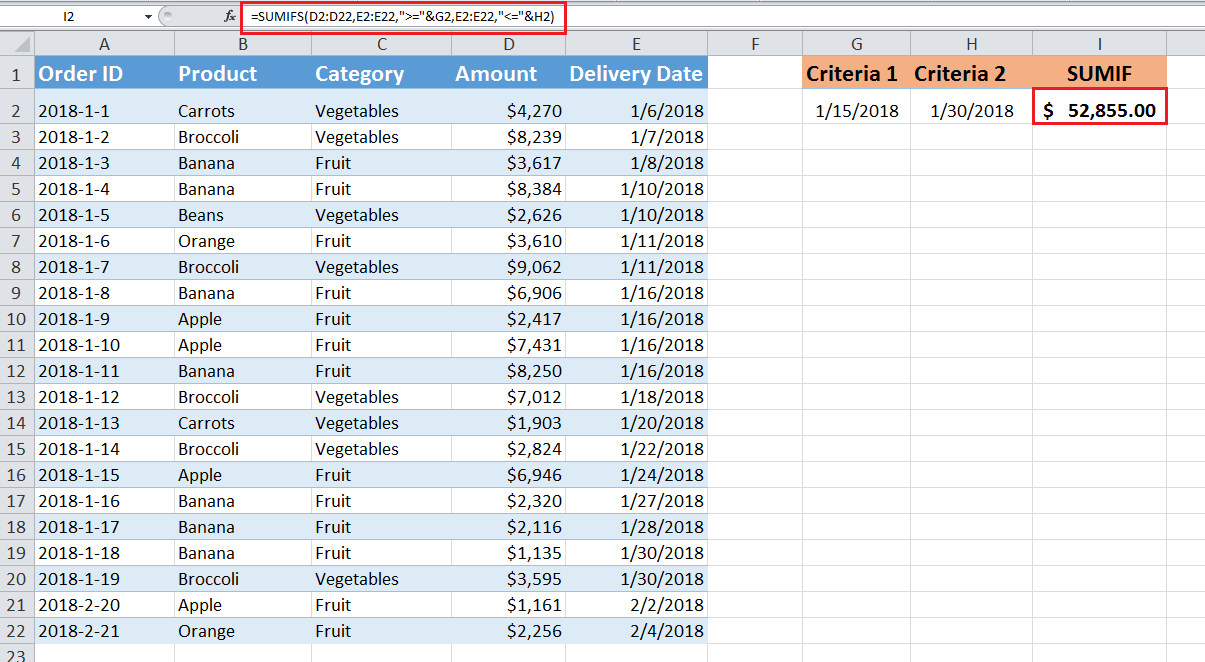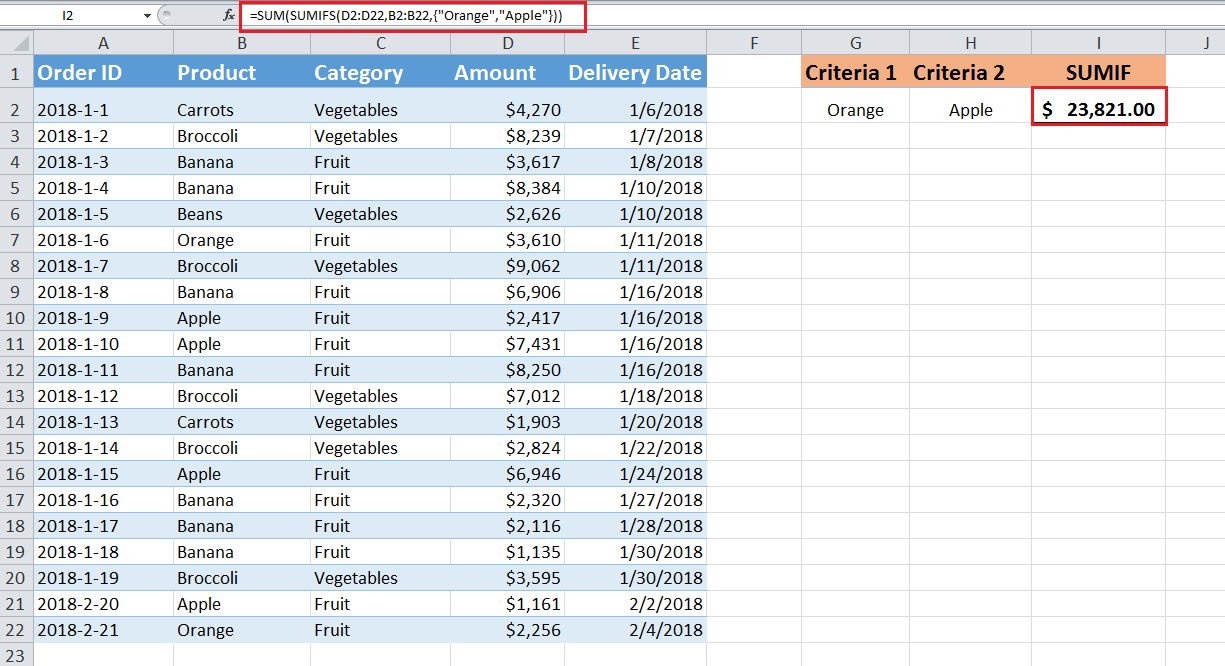Get instant live expert help with Excel or Google Sheets“My Excelchat expert helped me in less than 20 minutes, saving me what would have been 5 hours of work!”

#### Post your problem and you’ll get Expert help in seconds.

Your message must be at least 40 characters
Our professional Expert are available now. Your privacy is guaranteed.

# How to Use SUMIF with Multiple Criteria in Excel

The SUMIF function is designed to sum numbers based on one criterion. By default, it considers one range, one criterion, and one sum_range as per its syntax. But if you want to add numbers based on multiple criteria then you have the following options to use.

## Use SUMIF with multiple criteria

• You add up multiple SUMIF functions based on OR logic, applied for each criterion separately.

You need to use SUMIFS function that is by default designed to sum numbers with multiple criteria, based on AND logic.

• You can also use SUMIFS function to sum number with multiple criteria, based on OR logic, with an array constant.

In this article, you will learn how to sum numbers based on multiple criteria by using three of these options. Let’s assume you have data set of sales orders for various products, and you want to sum order amounts with multiple criteria.

### SUMIF function with multiple criteria based on OR logic

If you want to add numbers that meet either of the criteria (OR logic) from multiple criteria then you need to sum up two or more SUMIF functions in a single formula. Suppose you want to sum order amounts for “Beans” and “Broccoli” products using OR logic then you need to sum up two SUMIF functions in a single formula using the following pattern;

`=SUMIF(range, criteria1, sum_range) + SUMIF(range, criteria2, sum_range)`

`=SUMIF(B2:B22,"Beans",D2:D22)+SUMIF(B2:B22,"Broccoli",D2:D22)`

OR

`=SUMIF(B2:B22,G2,D2:D22)+SUMIF(B2:B22,H2,D2:D22)`### SUMIFS function with multiple criteria based on AND logic

If you want to sum numbers from a range when all of the specified criteria are met, based on AND logic, then you need to use SUMIFS function. It is important to know that all of the criteria must be met on single or multiple ranges to sum up numbers from sum_range.

The syntax of SUMIFS is;

`SUMIFS(sum_range, criteria_range1, criteria1, criteria_range2, criteria2,...)`

Suppose, you want to sum the orders’ amounts that are delivered between two dates then you will use SUMIFS function. Here you need to supply two criteria on the same range and where both of these criteria are met SUMIFS function sums those orders’ amounts.

`=SUMIFS(D2:D22,E2:E22,">="&G2,E2:E22,"<="&H2)`

Here, you have supplied two dates as criteria in cell references G2 and H2 along with expression “>=” and “<=”.### SUMIFS function with multiple criteria based on OR logic

As SUMIFS function by default entertains multiple criteria based on AND logic, but to sum numbers based on multiple criteria using OR logic, you need to SUMIFS function within an array constant.

An array constant is a set of multiple criteria provided in curly braces {} in a formula, like

{“criteria1”,”criteria2”,”criteria3”}

Array constant using OR logic forces SUMIFS function to sum numbers based on either of the multiple criteria in an array result and finally SUMfunction add up those array results, like;

`=SUM(SUMIFS(sum_range, criteria_range , {“criteria1”,”criteria2”,”criteria3”}))`

`=SUM({value1, value2, value3})`

Suppose you want to sum orders’ amounts for either of the products “Orange” and “Apple” supplied as criteria in array constant then you need to provide multiple criteria in SUMIFS function as follows;

`=SUM(SUMIFS(D2:D22,B2:B22,{"Orange","Apple"}))`

Remember, you cannot use an expression or cell reference an array constant.

Here, array constant forces SUMIFS function to generate the result in an array, like the following;

`=SUM ({5866, 17955})`

`=23,821`

Finally, SUM function adds up these array results to come up with total figure as shown below;Still need some help with Excel formatting or have other questions about Excel? Connect with a live Excel expert here for some 1 on 1 help. Your first session is always free.

### Did this post not answer your question? Get a solution from connecting with the expert.Another blog reader asked this question today on Excelchat:
Related blogs## Subscribe to Excelchat.coAnother blog reader asked this question today on Excelchat: